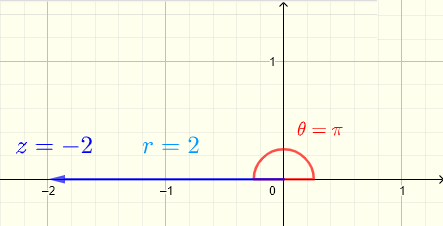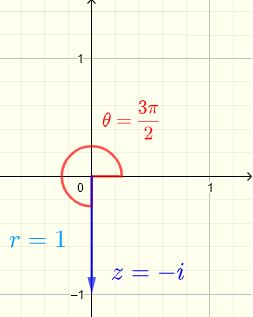# Complex Numbers in Exponential Form

Complex numbers are written in exponential form . The multiplications, divisions and power of complex numbers in exponential form are explained through examples and reinforced through questions with detailed solutions.

## Exponential Form of Complex Numbers

A complex number in standard form $$z = a + ib$$ is written in polar form as $z = r (\cos(\theta)+ i \sin(\theta))$ where $$r = \sqrt{a^2+b^2}$$ is called the modulus of $$z$$ and $$tan (\theta) = \left (\dfrac{b}{a} \right)$$ , such that $$0 \le \theta \lt 2\pi$$ , $$\theta$$ is called argument Examples and questions with solutions. of $$z$$
The graphical interpretations of $$a$$ , $$b$$ , $$r$$ and $$\theta$$ are shown below for a complex number on a complex plane.We now use
Euler's formula given by $$\displaystyle e^{i\theta} = \cos \theta + i \sin \theta$$ to write the complex number $$z$$ in exponential form as follows: $z = r e^{i\theta}$ where $$r$$ and $$\theta$$ as defined above.

Example 1
Plot the complex number $$z = -1 + i$$ on the complex plane and write it in
exponential form .
Solution to Example 1
The complex number $$z = -1 + i = a + i b$$ with $$a = -1$$ being the real part and $$b = 1$$ being the imaginary part, is plotted as a vector on a complex plane shown below. It is a vector whose components are the real part $$a$$ along the "real axis" and the imaginary part $$b$$ along the "imaginary axis". The argument angle $$\theta$$ is the angle in counterclockwise direction with initial side starting from the positive real part axis. The modulus $$r = \sqrt {a^2+b^2} = \sqrt {(-1)^2+(1)^2} = \sqrt 2$$ is the length of the vector.$$z = -1 + i = a + i b$$
gives $$a = - 1$$ and $$b = 1$$
$$r = \sqrt {a^2 + b^2} = \sqrt {(-1)^2 + 1^2} = \sqrt {1 + 1} = \sqrt 2$$
We first need to
find the reference angle $$\theta_r$$ which is the acute angle between the terminal side of $$\theta$$ and the real part axis.
$$\theta_r = \tan^{-1}\left|\dfrac{b}{a}\right| = \tan^{-1}\left|\dfrac{1}{-1}\right| =\tan^{-1} (1) = \dfrac{\pi}{4}$$
The real part of $$z$$ is negative and its imaginary part is posiive, hence the terminal side of $$\theta$$ is in quadrant II (see plot of $$z$$ above).
$$\theta$$ is computed as follows:
$$\theta = \pi - \theta_r = \pi - \dfrac{\pi}{4} = \dfrac{3\pi}{4}$$
$$z$$ in
exponential form is given by
$$z = \sqrt2 e^{i\dfrac{3\pi}{4}}$$

Example 2
a) Plot the complex numbers : $$i , - 2 , - i , - 1 - 2i$$ and $$1 - i$$ on the same complex plane.
b) Plot in separate complex planes and write the complex numbers : $$i , - 2 , - i , - 1 - 2i$$ and $$1 - i$$ in
exponential form .

Solution to Example 2
a) The plot all given complex numbers in the same complex plane is shown below.b) Plot and write in
exponential forms .
Let $$z = i = a + i b$$
gives $$a = 0$$ and $$b = 1$$
$$r = \sqrt {0^2 + 1^2} = 1$$
$$tan \theta = \dfrac{1}{0} = undefined$$
An angle whose tangent is undefined is an angle with terminal side on the imaginary axis.
In fact it is easier to determine $$\theta = \dfrac{\pi}{2}$$ from the plot of $$z = i$$ shown below.
Write in
exponential form : $$\quad z = i = r e^{i\theta} = e^{i\pi/2}$$Let $$z = -2 = a + i b$$
gives $$a = -2$$ and $$b = 0$$
$$r = \sqrt {(-2)^2 + 0^2} = 2$$
$$tan \theta = \dfrac{0}{-2} = 0$$
An angle whose tangent is equal to 0 is an angle with terminal side on the real axis.
It is easier to determine $$\theta = \pi$$ from the plot of $$z = -2$$ shown below.
Write in
exponential form : $$\quad z = -2 = r e^{i\theta} = 2 e^{i\pi}$$Let $$z = - i = a + i b$$
gives $$a = 0$$ and $$b = - 1$$
$$r = \sqrt {0^2 + (-1)^2} = 1$$
$$tan \theta = \dfrac{-1}{0} = undefined$$
An angle whose tangent is undefined is an angle with terminal side on the imaginary axis.
We determine $$\theta = \dfrac{3\pi}{2}$$ from the plot of $$z = - i$$ shown below.
Write in
exponential form : $$\quad z = - i = r e^{i\theta} = e^{ i 3\pi/2}$$Let $$z = - 1 -2i = a + i b$$
gives $$a = -1$$ and $$b = - 2$$
$$r = \sqrt {(-1)^2 + (-2)^2} = \sqrt 5$$
First find reference angle: $$tan \theta_r = |\dfrac{-2}{-1}| = 2$$ , $$\theta_r = \arctan 2$$
$$\theta = \pi + \arctan 2 \approx 4.25$$
Write in
exponential form : $$\quad z = - 1 -2i = r e^{i\theta} = \sqrt 5 e^{i (\pi + \arctan 2)}$$Let $$z = 1 - i = a + i b$$
gives $$a = 1$$ and $$b = - 1$$
$$r = \sqrt {(1)^2 + (-1)^2} = \sqrt 2$$
First find reference angle: $$tan \theta_r = |\dfrac{-1}{1}| = 1$$ , $$\theta_r = \dfrac{\pi}{4}$$
$$\theta = 2\pi - \theta_r = 2\pi - \dfrac{\pi}{4} = \dfrac{7\pi}{4}$$
Write in
exponential form : $$\quad z = 1 - i = r e^{i\theta} = \sqrt 2 e^{i ( 7 \pi/4)}$$Example 3
Write complex number $$z = \sqrt2 e^{ i \dfrac{5\pi}{4}}$$ in standard form.
Solution to Example 3
$$z = \sqrt2 e^{ i \dfrac{5\pi}{4}}$$
Use Euler's formula
$$= \sqrt2(\cos(\dfrac{5\pi}{4})+ i \sin(\dfrac{5\pi}{4}))$$
Simplify
$$= \sqrt2(-\dfrac{\sqrt2}{2} - i \dfrac{\sqrt2}{2})$$
$$= - 1 - i$$

Complex numbers in exponential form are easily multiplied and divided. The power and root of complex numbers in exponential form are also easily computed

## Multiplication of Complex Numbers in Exponential Forms

Let $$z_1 = r_1 e^{ i \theta_1}$$ and $$z_2 = r_2 e^{ i \theta_2}$$ be complex numbers in exponential form .
The product of $$z_1$$ and $$z_2$$ is given by
$z_1 z_2 = r_1 r_2 e ^{ i (\theta_1+\theta_2) }$

Example 3
Given $$z_1 = 3 e^{ i \pi/4 }$$ and $$z_2 = 5 e^{i 3\pi/4 }$$
Find $$z_1 z_2$$ and write it in standard form.
Solution to Example 3
$$z_1 z_2 = (3 e^{ i \pi/4 }) (5 e^{ i 3 \pi/4 } )$$
Multiply the modulii $$3$$ and $$5$$ together and apply exponent rule apply the rule of exponents $$e^x e^y = e^{x+y}$$
$$= (3 \times 5) e^{ i\pi/4 + i 3\pi/4 }$$
Simplify
$$= 15 e^{ i \pi }$$
Rewrite in polar form
$$= 15 (\cos \pi + i \sin \pi)$$
Simplify
$$= - 15$$

## Division of Complex Numbers in Exponential Forms

Let $$z_1 = r_1 e^{ i \theta_1}$$ and $$z_2 = r_2 e^{ i \theta_2 }$$ be complex numbers in exponential form .
The ratio (or division) of $$z_1$$ and $$z_2$$ is given by
$\dfrac{z_1}{ z_2} = \dfrac{r_1}{r_2} e ^{ i (\theta_1-\theta_2) }$

Example 4
Given $$z_1 = 10 e^{ i \pi/3 }$$ and $$z_2 = 2 e^{ i 2\pi/3 }$$
Find the product $$\dfrac{z_1}{z_2}$$ and write it in standard form.
Solution to Example 4
$$\dfrac{z_1}{z_2} = \dfrac{10 e^{ i \pi/3 }} {2 e^{ i 2 \pi/3 } }$$
Divide the modulii $$10$$ by $$2$$ and apply the rule of exponents $$\dfrac{e^x}{e^y} = e^{x-y}$$
$$= \dfrac {10}{2} e^{ i ( \pi/3 - 2\pi/3 ) }$$
$$= 5 e^{ - i \pi/3 }$$
Rewrite in polar form
$$= 5 (\cos (- \pi/3) + i \sin (- \pi/3) )$$
Simplify
$$= 5(1/2 - \dfrac{\sqrt 3}{2} i) = \dfrac{5}{2} - \dfrac{5\sqrt3}{2} i$$

You may also review
De Moivre's Theorem Power and Root of Complex Numbers.

## Questions

1) Write the following complex numbers in exponential forms .
1. $$z_1 = - 1$$
2. $$z_2 = - 2 i$$
3. $$z_3 = -\sqrt3 - i$$
4. $$z_4 = - 3 + 3\sqrt 3 i$$
5. $$z_5 = 7 - 7 i$$
.

2) Use the results in part a) above to evaluate the following expressions and write them in
exponential form .
1. $$z_4 z_5$$
2. $$\dfrac{z_3 z_5}{z_4}$$
.

## Solutions to the Above Questions

1)
1. $$z_1 = -1 = e^{ i \pi}$$
2. $$z_2 = - 2 i = 2 e^{ i 3\pi/2 }$$
3. $$z_3 = -\sqrt3 - i = 2 e^{ i 7\pi/6}$$
4. $$z_4 = - 3 + 3\sqrt 3 i = 6 e^{ i 2\pi/3 }$$
5. $$z_5 = 7 - 7 i = 7 \sqrt 2 e^{ i 7\pi/4}$$
.

2)
1. $$z_4 z_5 = (6 e^{ i 2\pi/3 }) (7 \sqrt 2 e^{ i 7\pi/4})$$
Use rule of multiplication given above
$$= 42 \sqrt 2 e^{i(2\pi/3 + 7\pi/4)}$$
Simplify
$$= 42 \sqrt 2 e^{i 29\pi/12}$$
Argument is larger than $$2 \pi$$ therefore you may subtract $$2 \pi$$ from it
$$= 42 \sqrt 2 e^{i (29\pi/12-2\pi )}$$
Simplify
$$= 42 \sqrt 2 e^{i 5\pi/12 }$$

2. $$\dfrac{z_3 z_5}{z_4} = \dfrac{( 2 e^{ i 7\pi/6})(7 \sqrt 2 e^{ i 7\pi/4})}{6 e^{ i 2\pi/3 }}$$
Use rule of multiplication and division given above
$$= \dfrac{2 \times 7 \sqrt 2}{6} e^{i(7\pi/6 + 7\pi/4 - 2\pi/3)}$$
Simplify
$$= \dfrac{7\sqrt{2}}{3} e^{i 9\pi/4}$$
Argument is larger than $$2 \pi$$ therefore you may subtract $$2 \pi$$ from it
$$= \dfrac{7\sqrt{2}}{3} e^{i (9\pi/4- 2\pi) }$$
Simplify
$$\dfrac{7\sqrt{2}}{3} e^{i \pi/4 }$$
.